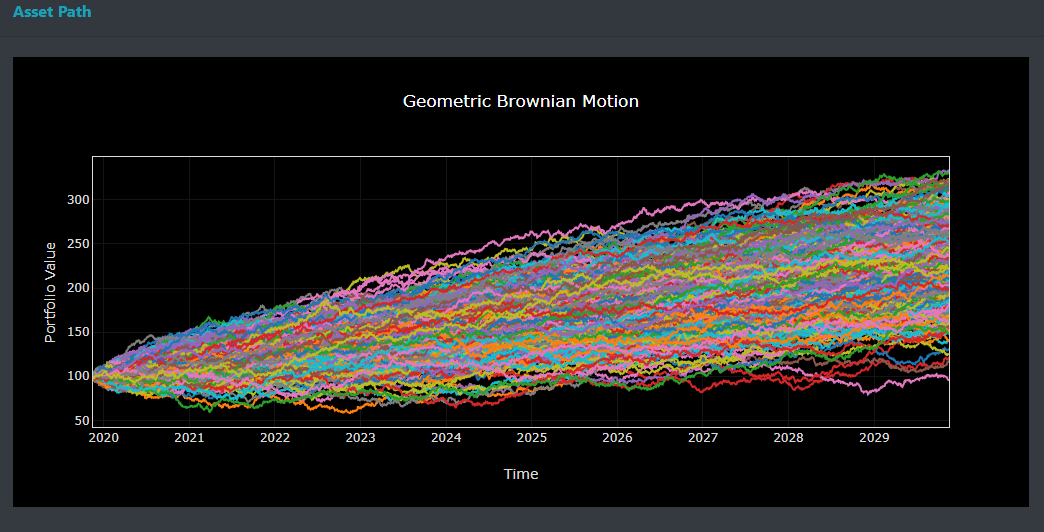## 13_R

### An analog of the CLT for stochastic process## 13_R assignament

### Request

An “analog” of the CLT for stochastic process: the standard Wiener process as “scaling limit” of a random walk and the functional CLT (Donsker theorem) or invariance principle. Explain the intuitive meaning of this result and how you have already illustrated the result in your homework.

### Donsker’s theorem

In probability theory, Donsker’s theorem (also known as Donsker’s invariance principle, or the functional central limit theorem), named after Monroe D. Donsker, is a functional extension of the central limit theorem.[1 ]

Let X1,X2,X3… be a sequence of independent and identically distributed random variables with mean 0 and variance 1. and lets take S:= (Sn) where Sn is the sum of all Xi

The stochastic process S is known as a random walk. Define the diffusively rescaled random walk (partial-sum process) by:The central limit theorem asserts that W^(n)(1) converges in distribution to a standard Gaussian random variable W(1) when n -> ∞.

Donsker’s invariance principle extends this convergence to the whole function W^(n):=hj(W^(n)(t) with t in 0,1So Donkers’s theorem states that as random varabile taken values in a Skorokhod space the random function W^(n) converges in distribution to a standard Brownian motion for n -> ∞.(Donsker’s invariance principle). This concept is used among the other field for assets price inference“url”,“https://en.wikipedia.org/wiki/Donsker%27s_theorem "